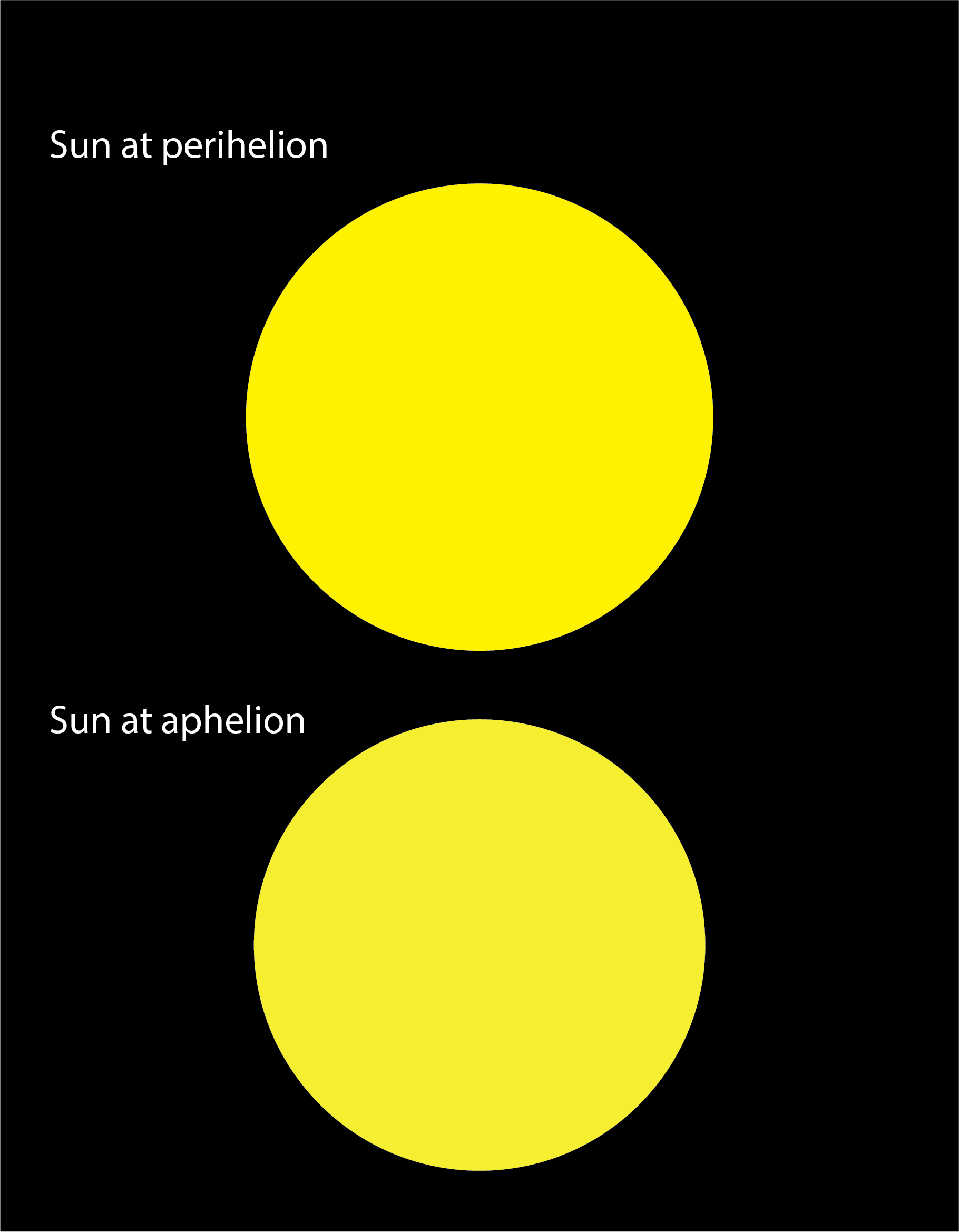# Changing Solar Distance

Between January 2 and 5 each year, the Earth reaches orbital perihelion, its closest distance to the Sun (0.983 AU).  Between July 3 and 6 each year, the Earth reaches orbital aphelion, its farthest distance from the Sun (1.017 AU).  These dates of perihelion and aphelion slowly shift across the calendar (always a half year apart) with a period between 22,000 and 26,000 years.

These distances can be easily derived knowing the semi-major axis (a) and orbital eccentricity (e) of the Earth’s orbit around the Sun, which are 1.000 and 0.017, respectively.

perihelion
q = a (1-e) = 1.000 (1-0.017) = 0.983 AU

aphelion
Q = a (1+e) = 1.000 (1+0.017) = 1.017 AU

So, the Earth is 0.034 AU closer to the Sun in early January than it is in early July.  This is about 5 million km or 3.1 million miles.

How great a distance is this, really?  The Moon in its orbit around the Earth is closer to the Sun around New Moon than it is around Full Moon.  Currently, this difference in distance ranges between 130,592 miles (April 2018) and 923,177 miles (October 2018).  Using the latter value, we see that the Moon’s maximum monthly range in distance from the Sun is 30% of the Earth’s range in distance from the Sun between perihelion and aphelion.

How about in terms of the diameter of the Sun?  The Sun’s diameter is 864,526 miles.  The Earth is just 3.6 Sun diameters closer to the Sun at perihelion than it is at aphelion.  Not much!  On average, the Earth is about 108 solar diameters distant from the Sun.

How about in terms of angular size?  When the Earth is at perihelion, the Sun exhibits an angular size of 29.7 arcminutes.  At aphelion, that angle is 28.7 arcminutes.Can you see the difference?